Chemical Reaction↪ When a chemical change occurs, a chemical reaction is said to have taken place.
↪ In other words, a chemical reaction causes chemical change in the reaction.
↪ Thus, we can say that both chemical change and reaction imply the same action.

⁕ Types of Chemical Reaction

↪ ﻿There are many types of chemical reactions. All the available reactions are broadly classified into different types according to their nature. They are:

1. Synthesis or Combination or Direct Union
↪ It is the union of two or more substances to form a single new substance.
For example: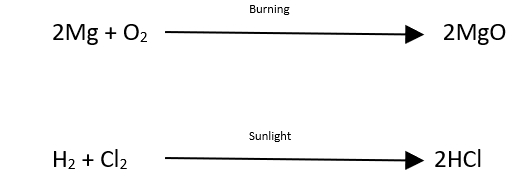2. Decomposition or Analysis
↪ It is the breaking up of a substance into simpler constituents by the application of heat, light, electricity etc.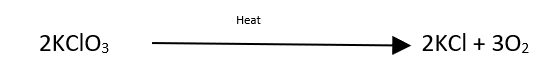3. Simple Displacement, Replacement or Substitution
↪ In this reaction, one element displaces another element from another compound.
For example: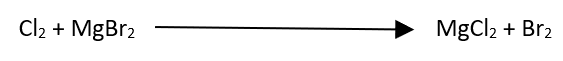4. Double Decomposition or Displacement or Mutual Exchange (Metathesis)
↪ It is the mutual exchange of radicals of two compounds taking part in the change and results in the formation of two new compounds.
This type of reaction is further classified as:

a. Acid-base reaction (Neutralization Reaction)
↪ It is process of formation of salt and water by the reaction between acid and base.
For example: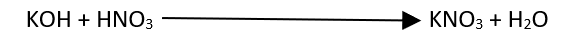b. Hydrolysis
↪ Any decomposition reaction which is brought about through the agency of water  are said to be hydrolysis.
For example: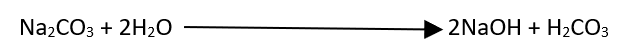c. Precipitation reaction
↪ It is a process of formation of one of the product as an insoluble product by mixing two compounds from their aqueous solution.
For example: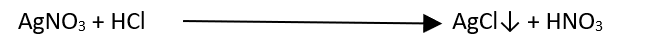d. Salt-acid displacement reaction
↪ It is also a double displacement reaction in which a salt of volatile acid interacts with non-volatile acid.
For example: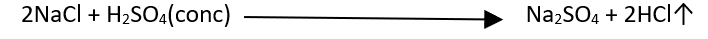⁕ How to balance a Chemical Equation
﻿↪ There is no need to memorize balanced chemical equation.
↪ Only reactant and product should be memorized in the form of skeleton equation.

The following methods for balancing a chemical equation are commonly used:

1. Hit and Trial Method
2. Partial Equation Method
3. Oxidation Number Method
4. Ion Electron Method

Methods number 3 and 4 are described in Oxidation and Reduction Chapter

⁕ Hit and trial method

Simple chemical equation are balanced by the so-called Hit and Trial method. It involves the following steps:
↪ ﻿Write the skeleton equation showing reactants at the left side and product at right.
↪ ﻿Write down the molecular formula of each substance.
↪ Count the number of atoms of each element on both sides of the equation.
↪ Then find the suitable numerical coefficients for the molecules of the reactants and products so that number of atoms of each element becomes equal.

⁕ Limitation of Hit and Trial Method

↪ It takes much of time to balance the equation.
↪ Hit and trial method of balancing equation containing more than two reactants are again more time consuming.

⁕ Partial Equation Method

↪ This method of balancing is applied in those reaction where many products are formed and their prediction by simple means is difficult.
To balance them by this method one should have the idea about:

a. Partial product formation

↪ Probable oxides and nascent O are assumed to produce and the partial equation is balanced by heat trial.

b. Nature of Oxides

↪ Metallic oxides
↪ Non-metallic oxides
↪ Neutral Oxides

c. Nascent H and nascent O formation

↪ Reactions via the formation O and H with HNO3 and elements are assumed to occur.

For Example: